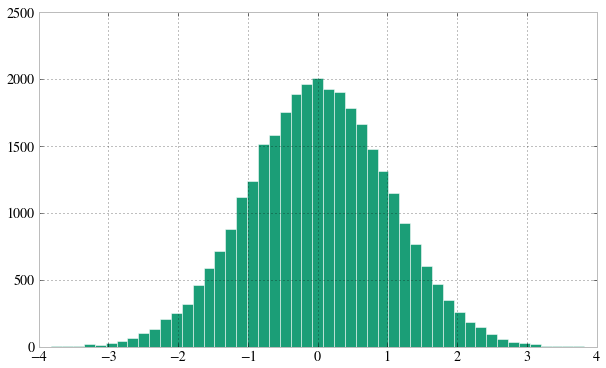## Lab6: Bayesianism, with MCMC.¶

In :
%matplotlib inline
from collections import defaultdict
import json

import numpy as np
import scipy as sp
import matplotlib.pyplot as plt
import pandas as pd

from matplotlib import rcParams
import matplotlib.cm as cm
import matplotlib as mpl

#colorbrewer2 Dark2 qualitative color table
dark2_colors = [(0.10588235294117647, 0.6196078431372549, 0.4666666666666667),
(0.8509803921568627, 0.37254901960784315, 0.00784313725490196),
(0.4588235294117647, 0.4392156862745098, 0.7019607843137254),
(0.9058823529411765, 0.1607843137254902, 0.5411764705882353),
(0.4, 0.6509803921568628, 0.11764705882352941),
(0.9019607843137255, 0.6705882352941176, 0.00784313725490196),
(0.6509803921568628, 0.4627450980392157, 0.11372549019607843)]

rcParams['figure.figsize'] = (10, 6)
rcParams['figure.dpi'] = 150
rcParams['axes.color_cycle'] = dark2_colors
rcParams['lines.linewidth'] = 2
rcParams['axes.facecolor'] = 'white'
rcParams['font.size'] = 14
rcParams['patch.edgecolor'] = 'white'
rcParams['patch.facecolor'] = dark2_colors
rcParams['font.family'] = 'StixGeneral'

def remove_border(axes=None, top=False, right=False, left=True, bottom=True):
"""
Minimize chartjunk by stripping out unnecesasry plot borders and axis ticks

The top/right/left/bottom keywords toggle whether the corresponding plot border is drawn
"""
ax = axes or plt.gca()
ax.spines['top'].set_visible(top)
ax.spines['right'].set_visible(right)
ax.spines['left'].set_visible(left)
ax.spines['bottom'].set_visible(bottom)

#turn off all ticks
ax.yaxis.set_ticks_position('none')
ax.xaxis.set_ticks_position('none')

#now re-enable visibles
if top:
ax.xaxis.tick_top()
if bottom:
ax.xaxis.tick_bottom()
if left:
ax.yaxis.tick_left()
if right:
ax.yaxis.tick_right()

pd.set_option('display.width', 500)
pd.set_option('display.max_columns', 100)


### The Bayesian formulation of regression and the posterior predictive distribution¶

(taken from Am207 class notes)

In the linear regression model we have data $D$, of $n$ observations
$D=\left\{ ({\bf x}_1, y_1), ({\bf x}_2,y_2), \ldots, ({\bf x}_n, y_n) \right\}$ where ${\bf x}$ denotes an input vector of dimension $D$ and $y$ denotes a scalar output (dependent variable). All data points are combined into a $D \times n$ matrix $X$. The model that determines the relationship between inputs and output is given by

$$y = \bf x^{T} {\bf w} + \epsilon$$

where ${\bf w}$ is a vector of parameters of the linear model. Usually there is a bias or offset is included, but for now we ignore it.

We assume that the additive noise $\epsilon$ is iid Gaussian with zero mean and variance $\sigma_n^2$ [ \epsilon \sim N(0, \sigma^2_n) ].

The likelihood is, because we assume independency, the product

\begin{eqnarray} L &=& p(\bf y|X,\bf w) = \prod_{i=1}^{n} p(y_i|\bf X_i, \bf w) = \frac{1}{\sqrt{2\pi}\sigma_n} \prod_{i=1}^{n} \exp{ \left( -\frac{(y_i-\bf X_i^T \bf w)^2}{2\sigma_n^2} \right)} \nonumber \\ &=& \frac{1}{\sqrt{2\pi}\sigma_n} \exp{\left( -\frac{| \bf y-X^T \bf w|^2 }{2\sigma_n^2} \right)} = N(X^T \bf w, \sigma_n^2 I) \end{eqnarray}

where $|x|$ denotes the Euclidean length of vector $\bf x$.

An alternative way of expressing the likelihood, which is convenient when sampling ${\bf w}$'s:

\begin{eqnarray} p(\bf y|\bf X,\bf w) &=& \frac{1}{\sqrt{2\pi}\sigma_n} \exp{\left( -\frac{| X^{-1} \bf y- \bf w|^2 \,\, (X^{-1} (X^{-1})^{T}) }{2\sigma_n^2} \right)} = N(X^{-1} \bf y, \sigma_n^2 (X^{-1} (X^{-1})^{T}) ) \end{eqnarray}

In the Bayesian framework inference we need to specify a prior over the parameters that expresses our belief about the parameters before we take any measurements. A wise choice is a zero mean Gaussian with covariance matrix $\Sigma$

\begin{equation} w \sim N(0, \Sigma) \end{equation}

We can now continue with the standard Bayesian formalism

\begin{eqnarray} p(\bf w| \bf y,X) &\propto& p(\bf y | X, \bf w) \, p(\bf w) \nonumber \\ &\propto& \exp{ \left(- \frac{1}{2 \sigma_n^2}(\bf y-X^T \bf w)^T(\bf y - X^T \bf w) \right)} \exp{\left( -\frac{1}{2} \bf w^T \Sigma^{-1} \bf w \right)} \nonumber \\ \end{eqnarray}

In the next step we complete the square' and obtain

\begin{equation} p(\bf w| \bf y,X) \propto \exp \left( -\frac{1}{2} (\bf w - \bar{\bf w})^T (\frac{1}{\sigma_n^2} X X^T + \Sigma^{-1})(\bf w - \bar{\bf w} ) \right) \end{equation}

where $\bar{\bf w} = \sigma_n^{-2}( \sigma_n^{-2}XX^T +\Sigma^{-1})^{-1} X \bf y$

This looks like a Gaussian and therefore the posterior is

$$p(\bf w| X, {\bf y}) \sim {\cal N}( \frac{1}{\sigma_n^2} A^{-1}Xy , A^{-1} )$$

where $A= \sigma_n^{-2}XX^T +\Sigma^{-1}$

To make predictions for a test case we average over all possible parameter predictive distribution values, weighted by their posterior probability. This is in contrast to non Bayesian schemes, where a single parameter is typically chosen by some criterion.

Thus the predictive distribution at some $x^{*}$ is given by averaging the output of all possible linear models w.r.t. the posterior

\begin{eqnarray} p(y^{*} | x^{*}, {\bf x,y}) &=& \int p({\bf y}^{*}| {\bf x}^{*}, {\bf w} ) p(\bf w| X, y)dw \nonumber \\ &=& {\cal N} \left(\frac{1}{\sigma_n^2} x^{*^{T}} A^{-1} X {\bf y}, x^{*^T}A^{-1}x^{*} \right), \end{eqnarray}

which is again Gaussian, with a mean given by the posterior mean multiplied by the test input and the variance is a quadratic form of the test input with the posterior covariance matrix, showing that the predictive uncertainties grow with the magnitude of the test input, as one would expect for a linear model.

### Bayesian as an updating of knowledge¶

In :
a0=-0.3
a1=0.5
N=20
noiseSD=0.2
priorPrecision=2.0
u=np.random.rand(20)
x=2.*u -1.
def randnms(mu, sigma, n):
return sigma*np.random.randn(n) + mu
y=a0+a1*x+randnms(0.,noiseSD,N)
plt.scatter(x,y)

Out:
<matplotlib.collections.PathCollection at 0x106038e50>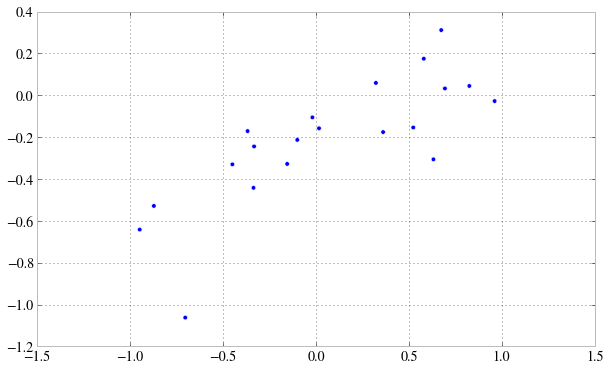In :
from scipy.stats import norm
likelihoodSD = noiseSD # Assume the likelihood precision, beta, is known.
likelihoodPrecision = 1./(likelihoodSD*likelihoodSD)

In :
from _multivariate import multivariate_normal

In :
def cplot(f):
plt.figure()
xx,yy=np.mgrid[-1:1:.01,-1:1:.01]
pos = np.empty(xx.shape + (2,))
pos[:, :, 0] = xx
pos[:, :, 1] = yy
ax=plt.contourf(xx, yy, f(pos))
#data = [x, y]
return ax

In :
priorMean = np.zeros(2)
priorSigma = np.eye(2)/priorPrecision #Covariance Matrix
priorPDF = lambda w: multivariate_normal.pdf(w,priorMean,priorSigma)
priorPDF([1,2])

Out:
0.0021447551423913074
In :
np.transpose(priorMean)

Out:
array([ 0.,  0.])
In :
cplot(priorPDF)

Out:
<matplotlib.contour.QuadContourSet instance at 0x107853998>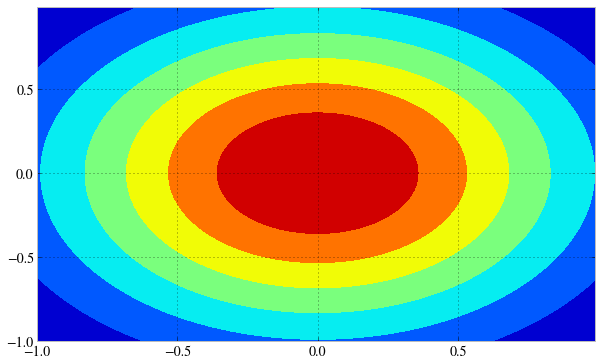In :
def plotSampleLines(mu, sigma, numberOfLines, dataPoints=None):
#Plot the specified number of lines of the form y = w0 + w1*x in [-1,1]x[-1,1] by
# drawing w0, w1 from a bivariate normal distribution with specified values
# for mu = mean and sigma = covariance Matrix. Also plot the data points as
# blue circles.
#print "datap",dataPoints
fig=plt.figure()

for i in range(numberOfLines):
w = np.random.multivariate_normal(mu,sigma)
func = lambda x: w + w*x
xx=np.array([-1,1])
plt.plot(xx,func(xx),'r')
if dataPoints:
plt.scatter(dataPoints,dataPoints)
ax=plt.gca()
ax.set_xlim([-1,1])
ax.set_ylim([-1,1])
plt.show()

In :
plotSampleLines(priorMean,priorSigma,6)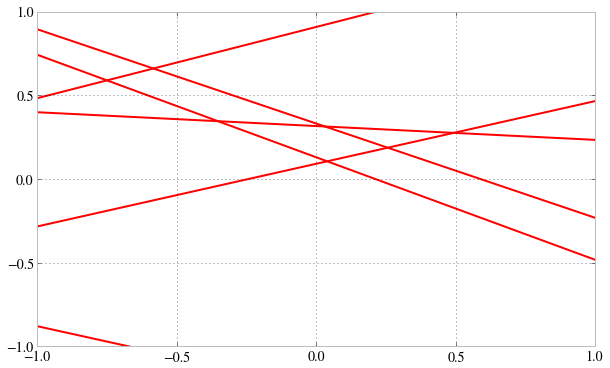In :
# Given the mean = priorMu and covarianceMatrix = priorSigma of a prior
# Gaussian distribution over regression parameters; observed data, xtrain
# and ytrain; and the likelihood precision, generate the posterior
# distribution, postW via Bayesian updating and return the updated values
# for mu and sigma. xtrain is a design matrix whose first column is the all
# ones vector.
def update(x,y,likelihoodPrecision,priorMu,priorSigma):
postSigmaInv  = np.linalg.inv(priorSigma) + likelihoodPrecision*np.outer(x.T,x)
postSigma = np.linalg.inv(postSigmaInv)
postMu = np.dot(np.dot(postSigma,np.linalg.inv(priorSigma)),priorMu) + likelihoodPrecision*np.dot(postSigma,np.outer(x.T,y)).flatten()
postW = lambda w: multivariate_normal.pdf(w,postMu,postSigma)
return postW,postMu,postSigma

In :
# For each iteration plot  the
# posterior over the first i data points and sample lines whose
# parameters are drawn from the corresponding posterior.
mu = priorMean
sigma = priorSigma
iterations=2
muhash={}
sigmahash={}
for i in [1,2,3,4,5,6,7,8,9,10,11,12,13,14,15,16,17,18,19]:
postW,mu,sigma = update(np.array([1,x[i]]),y[i],likelihoodPrecision,mu,sigma)
muhash[i]=mu
sigmahash[i]=sigma
if i in [1,4,7,10,19]:
cplot(postW)
#plotSampleLines(mu,sigma,6,(x[0:i],y[0:i]))
#print "=========", i, x[0:i],y[0:i]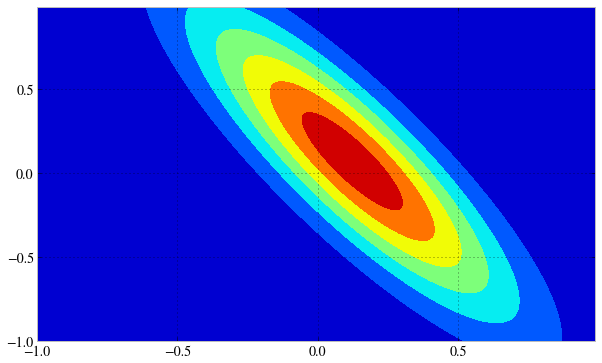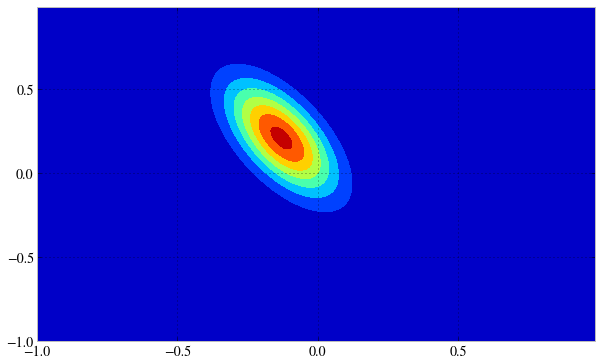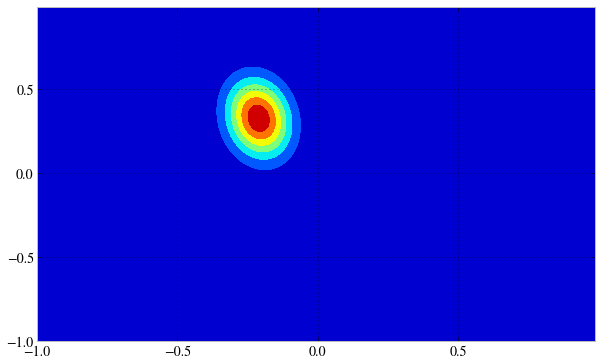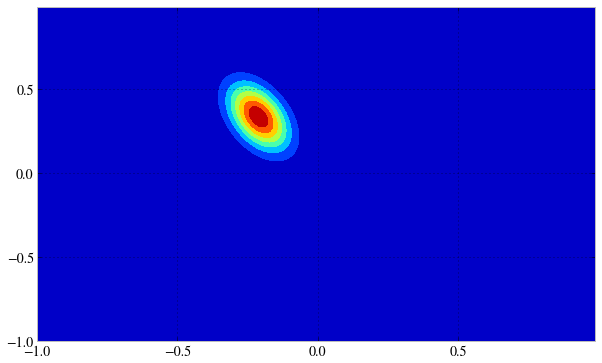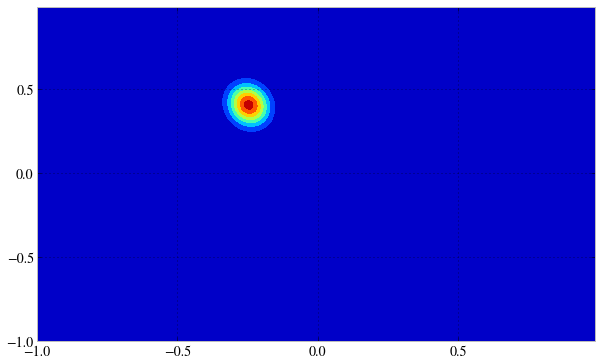In :
for i in [1,2,3,4,5,6,7,8,9,10,11,12,13,14,15,16,17,18,19]:
if i in [1,4,7,10,19]:
plotSampleLines(muhash[i],sigmahash[i],6, (x[0:i],y[0:i]))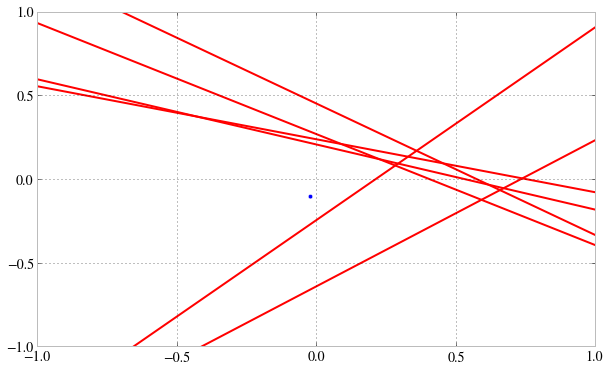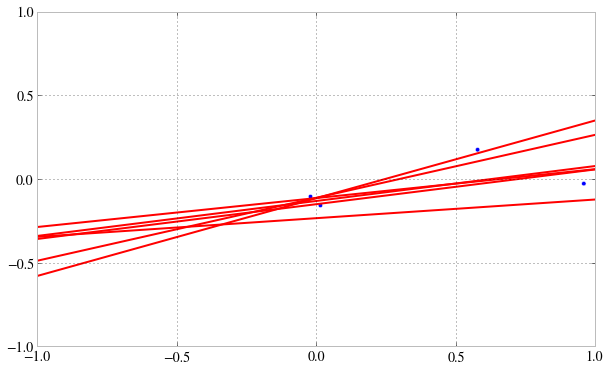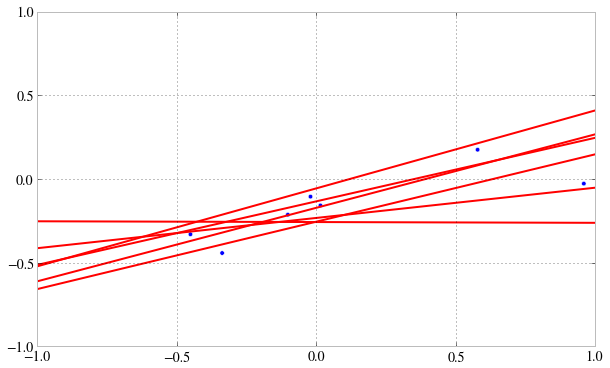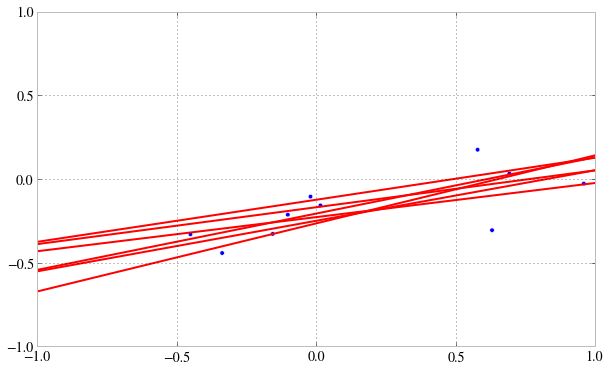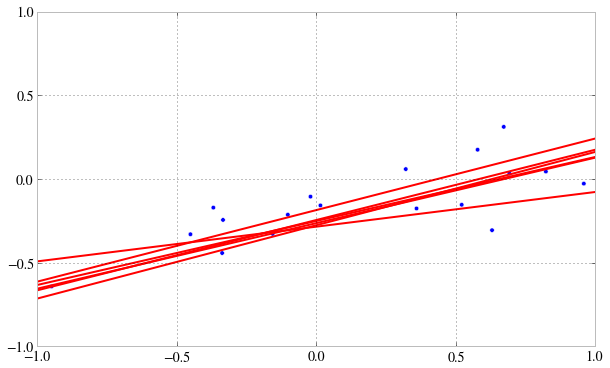### MCMC sampling¶

Monte Carlo sampling is design to estimate various characteristics of a distribution such as the mean, variance, kurtosis, or any other statistic.

Markov chains involve a stochastic sequential process where we can sample states from some stationary distribution. The goal of MCMC is to design a Markov chain such that the stationary distribution of the chain is exactly the distribution that we are interesting in sampling from. This is called the target distribution.

In other words, the states sampled from the Markov chain should follow the same statistics of samples drawn from the target distribution. The idea is to use some clever methods for setting up the proposal distribution such that no matter how we initialize each chain, we will convergence to the target distribution.

### MCMC, MH Sampler¶

The Metropolis-Hastings (MH) algorithm is the most popular of the MCMC methods (Metropolis et al 1953, Hastings 1970). As a matter of fact we will see that most MCMC algorithms can be interpreted as special cases of this algorithm.

Suppose our goal is to sample from the target density $p(x)$, with $-\infty< x < \infty$ . The Metropolis-Hastings sampler creates a Markov chain that produces a sequence of values:

$$x^{(1)} \rightarrow x^{(2)} \rightarrow \ldots \rightarrow x^{(t)} \ldots$$

where $x^{(t)}$ represents the state of a Markov chain at iteration $t$. The samples from the chain, after burnin, reflect samples from the target distribution $p(x)$.

The MH algorithm, initializes the first state, $x^{(1)}$ to some initial value, and then uses a proposal distribution $q(x_s|x_t)$ to sample a candidate value $x_s$ given the current value of $x_t$ according to $q(x_s|x_t)$. The Markov chain then moves towards $x_s$ with the acceptance probability

$$A(x_t,x_s) = \min\left[1, \frac{p(x_s) \, q(x_t|x_s)}{p(x_t) q(x_s|x_t) } \right]$$

otherwise it remains at $x_t$.

### M to be precise.¶

Note that if the transition probability is symmetric $q(x_s|x^{(i)}) = q(x^{(i)}|x_s)$ then the acceptance probability becomes

$$A(x,x_s) = \min\left[1, \frac{p(x_s)}{p(x) } \right]$$

which was the original algorithm proposed by Metropolis, thus the Metropolis algorithm.

A major advantage of the MH sampler is that equation involves only a ratio of densities. Therefore, any terms independent of $x$ in the functional form of $p(x)$ will drop out. We do not need to know the normalizing constant of the density or probability. The fact that this procedure allows us to sample from unnormalized distributions is one of its major attractions. Sampling from unnormalized distributions frequently happens in Bayesian models, where calculating the normalization constant is difficult or impractical.

The MH algorithm is very simple, but it requires careful design of the proposal distribution $q(x_s| x)$.

Later we will see that many MCMC algorithms arise by considering specific choices of this distribution.
A way to design these sampling mechanism is to ensure that detailed balance is satisfied. However, it is also important to design samplers that converge quickly or the burnin time is short.

In :
Nmc=200000
burnin=40000
t1 = np.zeros(Nmc)
i=0
#Try 0.1, 1.0, 10.0, 100.0
sig=1.
t1_prev=np.random.randn()
print "Init",t1_prev

def randnms(mu, sigma):
return sigma*np.random.randn() + mu

posterior = lambda x: np.exp(-x*x/2.)

proposal=randnms

while i < Nmc:
#proposal
t1_star=proposal(t1_prev, sig)
#New Posterior
P_star=posterior(t1_star)
#Old Posterior
P_prev=posterior(t1_prev)

A=np.min([P_star/P_prev, 1.0])
U=np.random.rand()

if U < A :#accept
t1[i]=t1_star
t1_prev=t1_star
else:#reject
t1[i]=t1_prev

i=i+1


Init 0.929786392743

In :
t1clean=t1[burnin:Nmc:5]
plt.plot(range(len(t1clean)),t1clean,'.')

Out:
[<matplotlib.lines.Line2D at 0x1085c4e90>]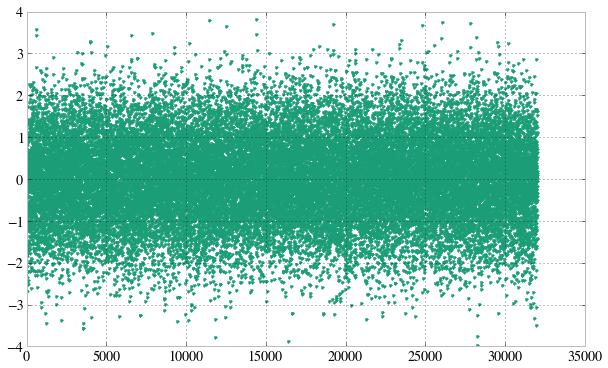In :
plt.acorr(t1clean-np.mean(t1clean), maxlags=100, lw=1 , normed=True);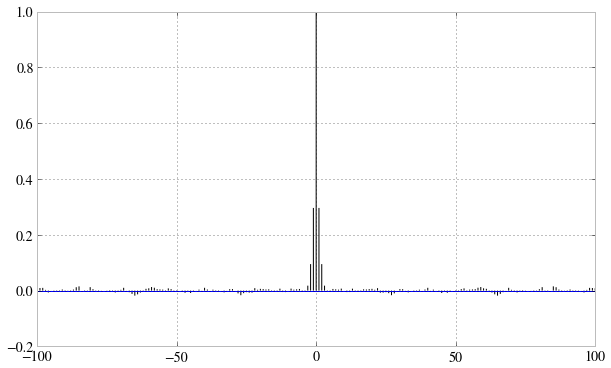In :
plt.hist(t1clean, bins=50);
`